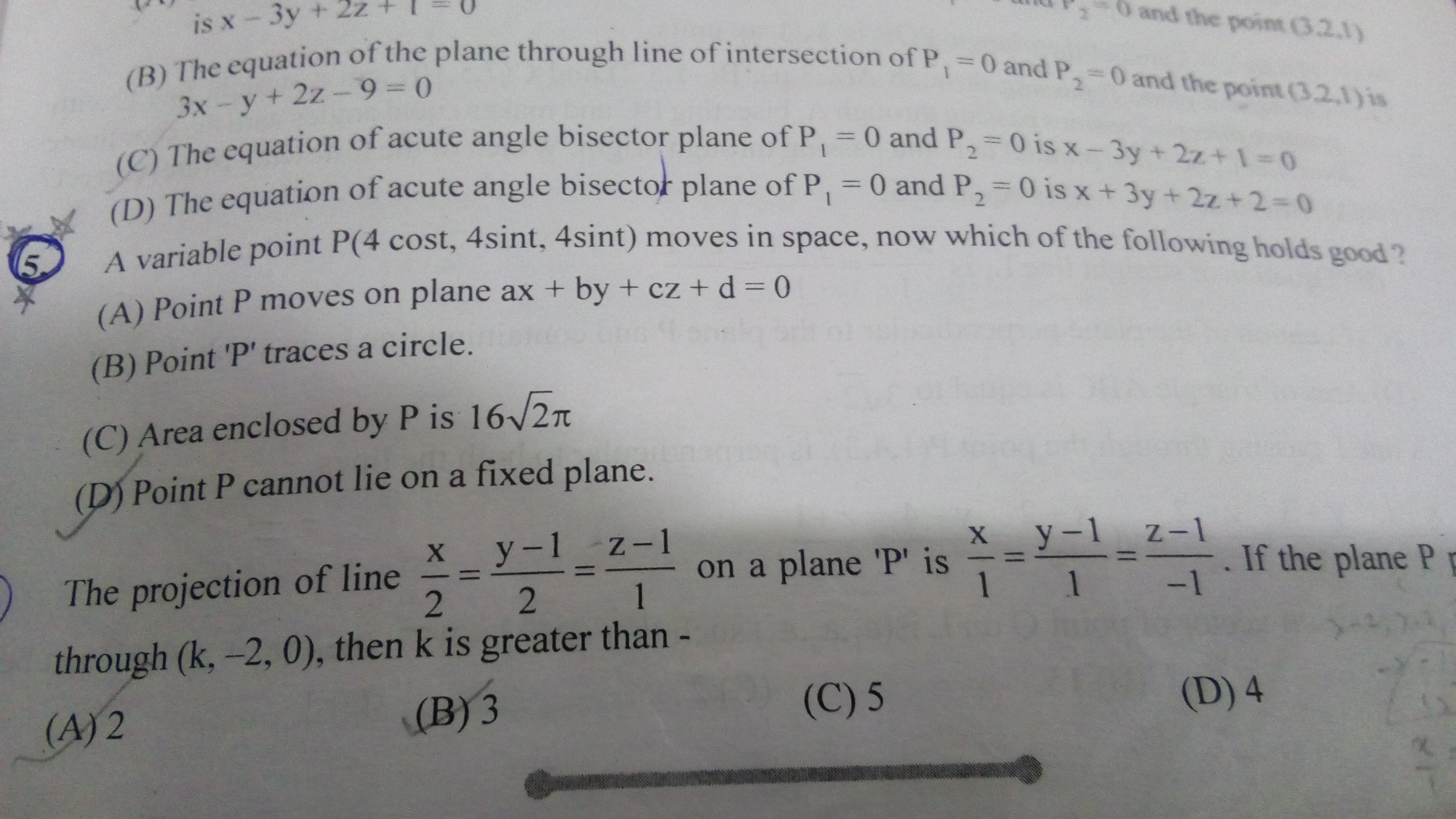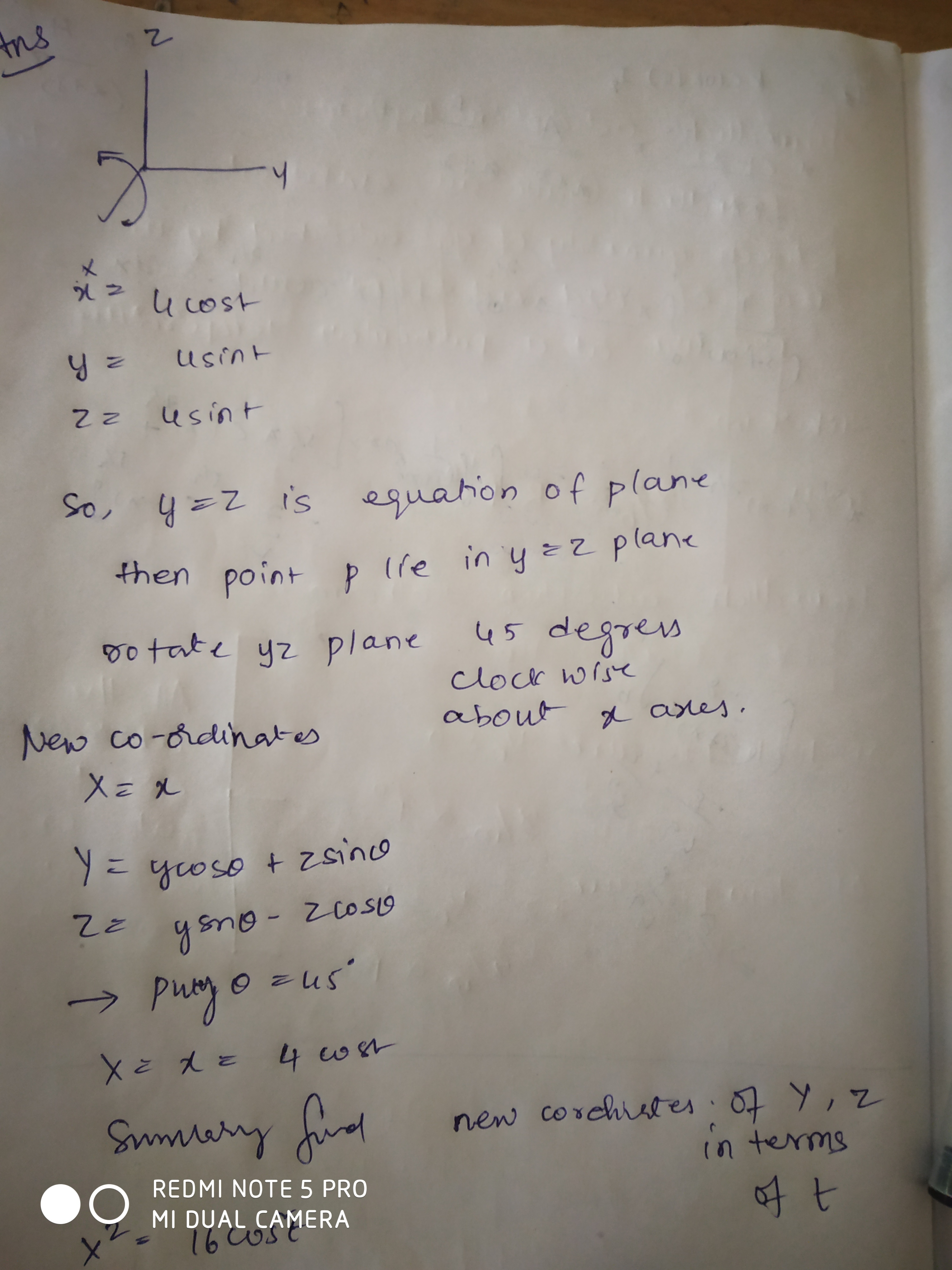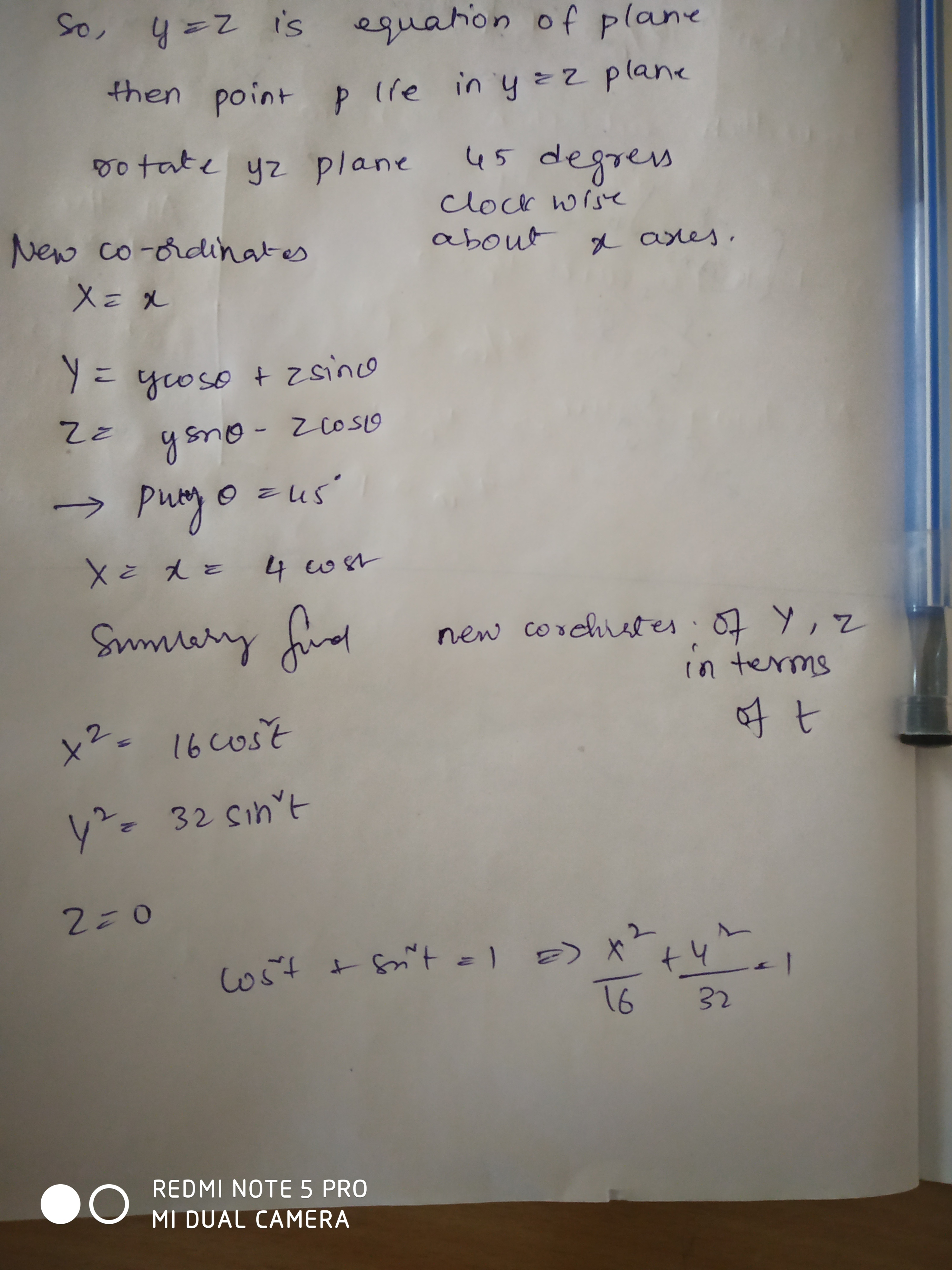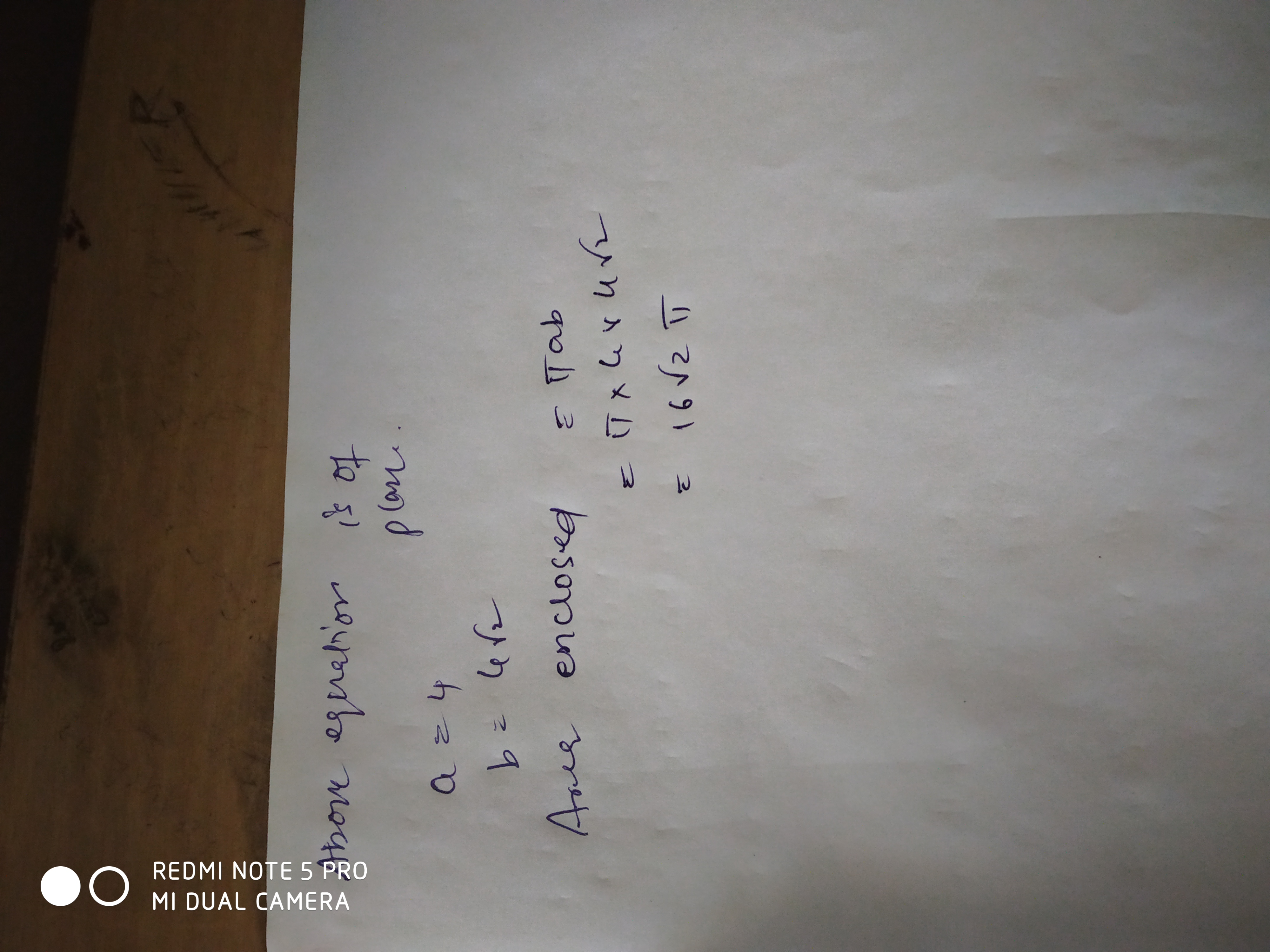# Doubt in 3d geometryPlz help in q no. 5
Ans is a and c

Here Locus is the Intersection of cylinder with the plane y=z.

It can be looked at as a intersection of 2 cylinders.

For calculation of Area...

P is an Ellipse in the plane y=z whose vertex is at (4,0,0)

Find the length of the major and minor axis by taking max. and min.

Distance from center.

Here x=4\cos t,y=4\sin t,z=4\sin t

Now eliminating t, we get 2x^2+y^2+z^2=32.

Which is equation of Ellipsoid and plane y=z.

Now variable point P move on plane formed by

Ellipsoid 2x^2+y^2+z^2=32 and y=z. (Just Draw it)

which is an ellipse whose center is at origin and length

of major axis length is 4\sqrt{2} and minor axis length is 4

So Area of that ellipse is \pi \cdot 4\sqrt{2}\cdot 4=16\sqrt{2} Sq. unit.

1 Like